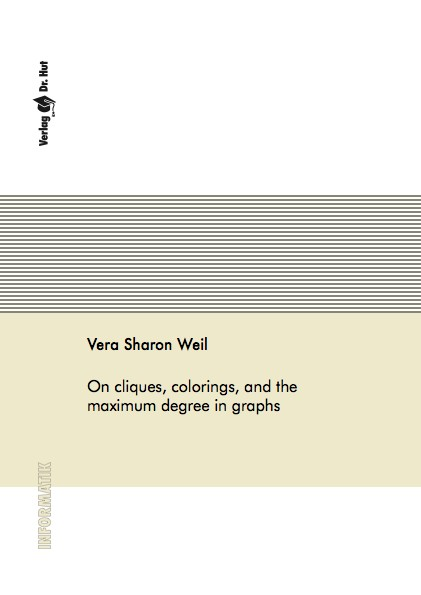Datenbestand vom 23. Oktober 2020aktualisiert am 23. Oktober 2020

# ISBN 978-3-8439-1992-060,00 € inkl. MwSt, zzgl. Versand

978-3-8439-1992-0, Reihe Informatik

Vera Sharon Weil
On cliques, colorings, and the maximum degree in graphs

119 Seiten, Dissertation Universität Köln (2015), Softcover, A5

## Zusammenfassung / Abstract

A graph parameter p is monotone if p(H)<= p(G) whenever H is an induced subgraph of a graph G. We investigate the relationship between the maximum degree Delta of a graph and other monotone graph parameters, with a focus on the clique number omega and the chromatic number chi. By a characterization of the minimal forbidden induced subgraphs, we describe the largest hereditary graph class where the maximum degree of each graph in this class is bounded by a monotonically increasing function on a monotone graph parameter on the graph. For omega and chi, we show that if a function on one of these graph parameters obeys a certain condition, the set of minimal forbidden induced subgraphs of the thereby defined hereditary graph class is finite. We prove that the largest hereditary graph classes where the maximum degree is bounded by a function on omega are exactly those where the maximum degree is bounded by a function on chi. For every k in N_0, we introduce the hereditary graph classes Omega_k and Upsilon_k, that is, the largest hereditary graph classes where the maximum degree is bounded by the function omega + k - 1 or by the function chi+k-1, respectively. For Omega_k and Upsilon_k, we provide the finite set of minimal forbidden induced subgraphs for every k. We compare Omega_k and Upsilon_k and subsequently characterize the graph class where the minimal forbidden induced subgraphs of Omega_k and Upsilon_k coincide for each k. Moreover, we provide some results on the chromatic number of the graphs in Omega_k and show that if Upsilon_k is restricted to claw-free graphs, then the minimal forbidden induced subgraphs can be described in terms of Tura'n graphs. In addition, the computational complexity of CLIQUE and K-COLORABILITY is investigated in the considered hereditary graph classes. Given a monotone graph parameter p, we say that a vertex in a graph is p-critical if removing the vertex from the graph produces a graph with lower p. We detect the graphs such that every connected induced subgraph of the graph contains a vertex that is p-critical given that p equals Delta, omega and chi, respectively. We suppose the existence of a vertex-minimal counterexample to a conjecture of Reed on chi, Delta and omega, reveal some structural properties of this counterexample and derive some graph classes for which the conjecture holds.